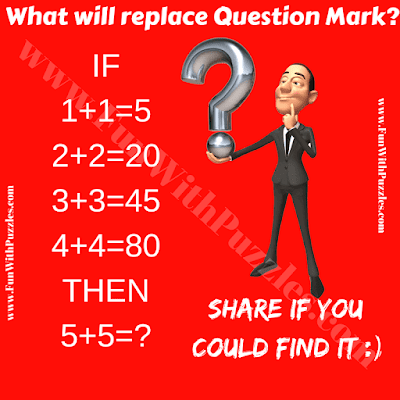Here is very simple Maths Picture Riddle which will give a twist to your brain. In this Maths Riddle, there are some number equations which are wrong Mathematically :(. However, the number on both side of these equations share for the formula which when applied to all the equations makes all these equations correct. Your challenge is to find this hidden Mathematical formula which will make these equations correct. Let's see how much time you will take to find the missing number in this Maths Picture Riddle?Can you solve this Maths Picture Riddle?

The answer to this "Maths Picture Riddle", can be viewed by clicking on the button. Please do give your best try before looking at the answer.

Unknown said...

125 A*B*5